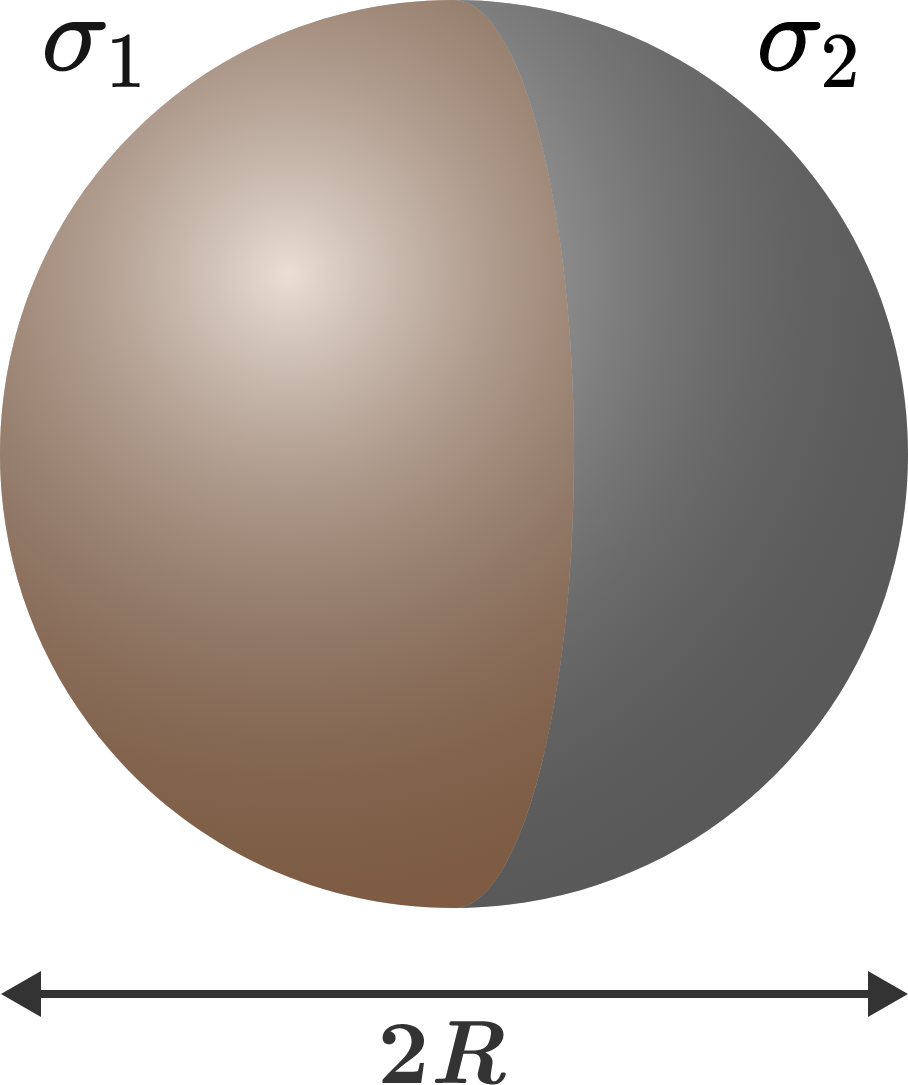# Force on one half due to the otherTwo half-spheres with fixed surface charge densities $\sigma_1$ and $\sigma_2$ and radius $R$ are brought into contact, as shown to the right.

Find the force of interaction between the two half-spheres.

×